## Absolute Value Functions

### Learning Outcomes

• Graph an absolute value function.
• Find the intercepts of an absolute value function.Distances in deep space can be measured in all directions. As such, it is useful to consider distance in terms of absolute values. (credit: “s58y”/Flickr)

Until the 1920s, the so-called spiral nebulae were believed to be clouds of dust and gas in our own galaxy some tens of thousands of light years away. Then, astronomer Edwin Hubble proved that these objects are galaxies in their own right at distances of millions of light years. Today, astronomers can detect galaxies that are billions of light years away. Distances in the universe can be measured in all directions. As such, it is useful to consider distance as an absolute value function. In this section, we will investigate absolute value functions.

## Understanding Absolute Value

Recall that in its basic form $\displaystyle{f}\left({x}\right)={|x|}$, the absolute value function, is one of our toolkit functions. The absolute value function is commonly thought of as providing the distance a number is from zero on a number line. Algebraically, for whatever the input value is, the output is the value without regard to sign.

### A General Note: Absolute Value Function

The absolute value function can be defined as a piecewise function

$f(x) =\begin{cases}x ,\ x \geq 0 \\ -x , x < 0\\ \end{cases}$

### Example: Determine a Number within a Prescribed Distance

Describe all values $x$ within or including a distance of 4 from the number 5.

### Try It

Describe all values $x$ within a distance of 3 from the number 2.

### Example: Resistance of a Resistor

Electrical parts, such as resistors and capacitors, come with specified values of their operating parameters: resistance, capacitance, etc. However, due to imprecision in manufacturing, the actual values of these parameters vary somewhat from piece to piece even when they are supposed to be the same. The best that manufacturers can do is to try to guarantee that the variations will stay within a specified range, often $\pm 1\%,\pm5\%,$ or $\displaystyle\pm10\%$.

Suppose we have a resistor rated at 680 ohms, $\pm 5\%$. Use the absolute value function to express the range of possible values of the actual resistance.

### Try It

Students who score within 20 points of 80 will pass a test. Write this as a distance from 80 using absolute value notation.

The most significant feature of the absolute value graph is the corner point at which the graph changes direction. This point is shown at the origin.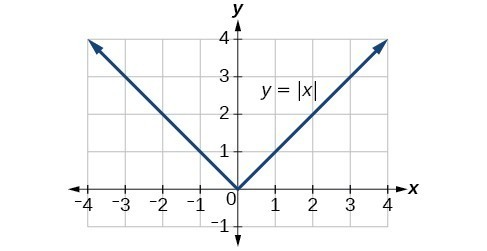The graph below is of $y=2\left|x - 3\right|+4$. The graph of $y=|x|$ has been shifted right 3 units, vertically stretched by a factor of 2, and shifted up 4 units. This means that the corner point is located at $\left(3,4\right)$ for this transformed function.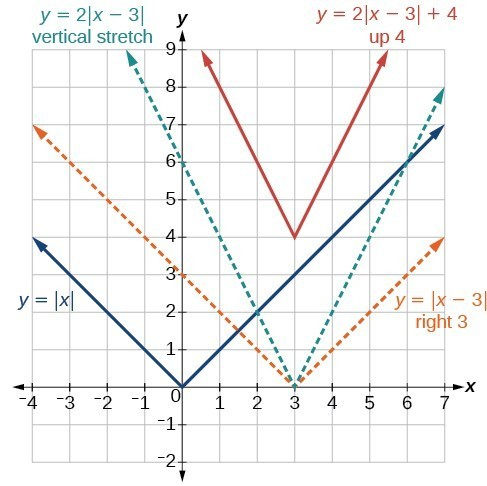### Example: Writing an Equation for an Absolute Value Function

Write an equation for the function graphed below.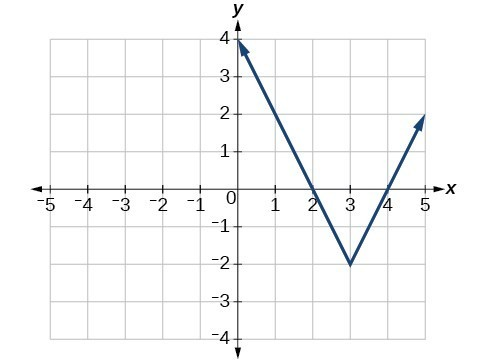Q & A

If we couldn’t observe the stretch of the function from the graphs, could we algebraically determine it?

Yes. If we are unable to determine the stretch based on the width of the graph, we can solve for the stretch factor by putting in a known pair of values for $x$ and $f\left(x\right)$.

$f\left(x\right)=a|x - 3|-2$

Now substituting in the point (1, 2)

$\begin{array}{l}2=a|1 - 3|-2\hfill \\ 4=2a\hfill \\ a=2\hfill \end{array}$

### Try It

Write the equation for the absolute value function that is horizontally shifted left 2 units, vertically flipped, and vertically shifted up 3 units.

Q & A

Do the graphs of absolute value functions always intersect the vertical axis? The horizontal axis?

Yes, they always intersect the vertical axis. The graph of an absolute value function will intersect the vertical axis when the input is zero.

No, they do not always intersect the horizontal axis. The graph may or may not intersect the horizontal axis depending on how the graph has been shifted and reflected. It is possible for the absolute value function to intersect the horizontal axis at zero, one, or two points.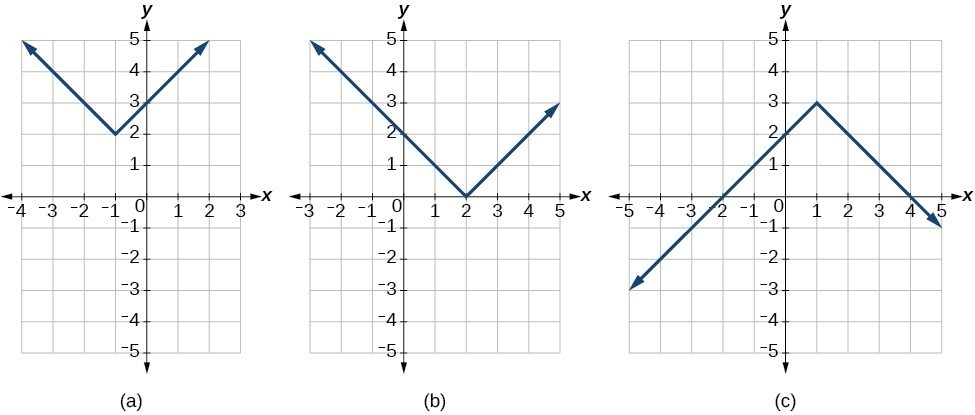(a) The absolute value function does not intersect the horizontal axis. (b) The absolute value function intersects the horizontal axis at one point. (c) The absolute value function intersects the horizontal axis at two points.

## Find the Intercepts of an Absolute Value Function

Knowing how to solve problems involving absolute value functions is useful. For example, we may need to identify numbers or points on a line that are at a specified distance from a given reference point.

### How To: Given the formula for an absolute value function, find the horizontal intercepts of its graph.

1. Isolate the absolute value term.
2. Use $|A|=B$ to write $A=B$ or $\mathrm{-A}=B$, assuming $B>0$.
3. Solve for $x$.

### Example: Finding the Zeros of an Absolute Value Function

For the function $f\left(x\right)=|4x+1|-7$ , find the values of $x$ such that $\text{ }f\left(x\right)=0$ .

### Try It

For the function $f\left(x\right)=|2x - 1|-3$, find the values of $x$ such that $f\left(x\right)=0$.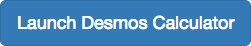## Contribute!

Did you have an idea for improving this content? We’d love your input.